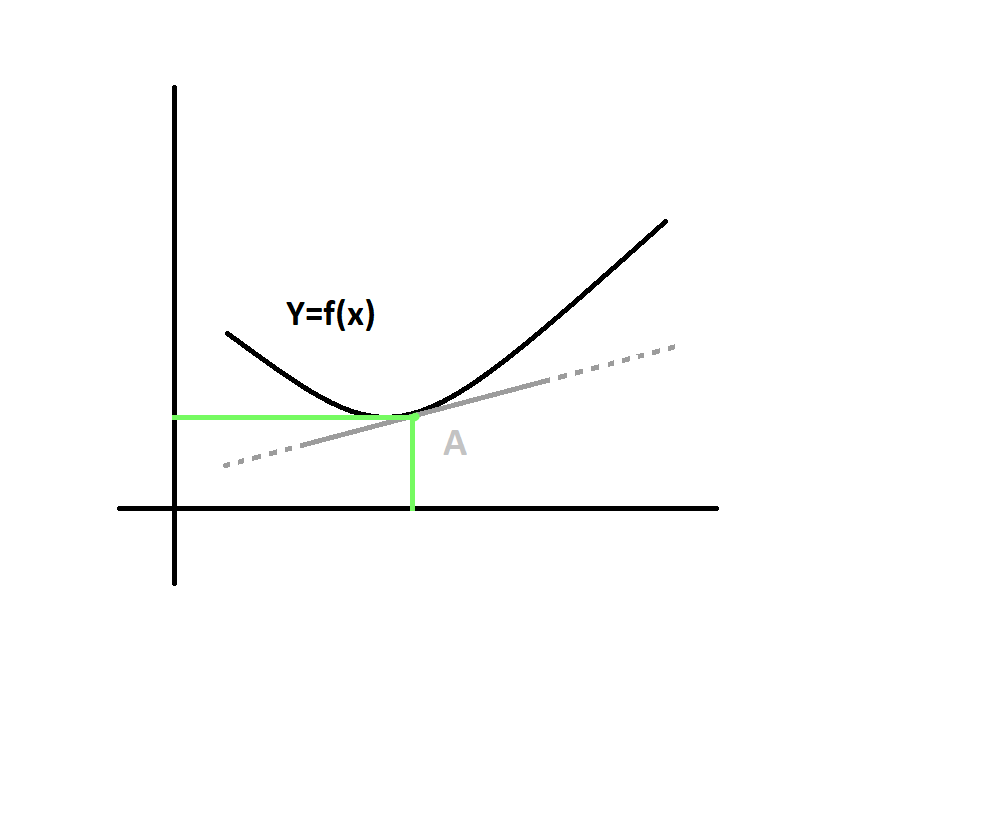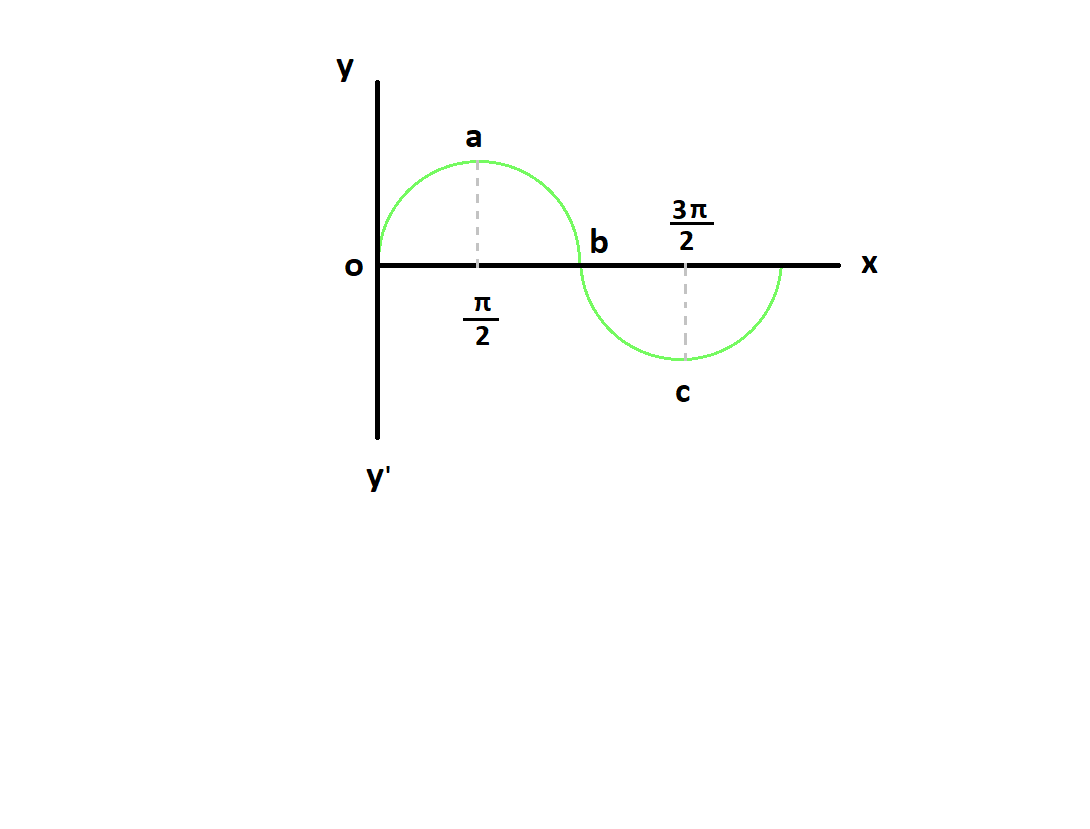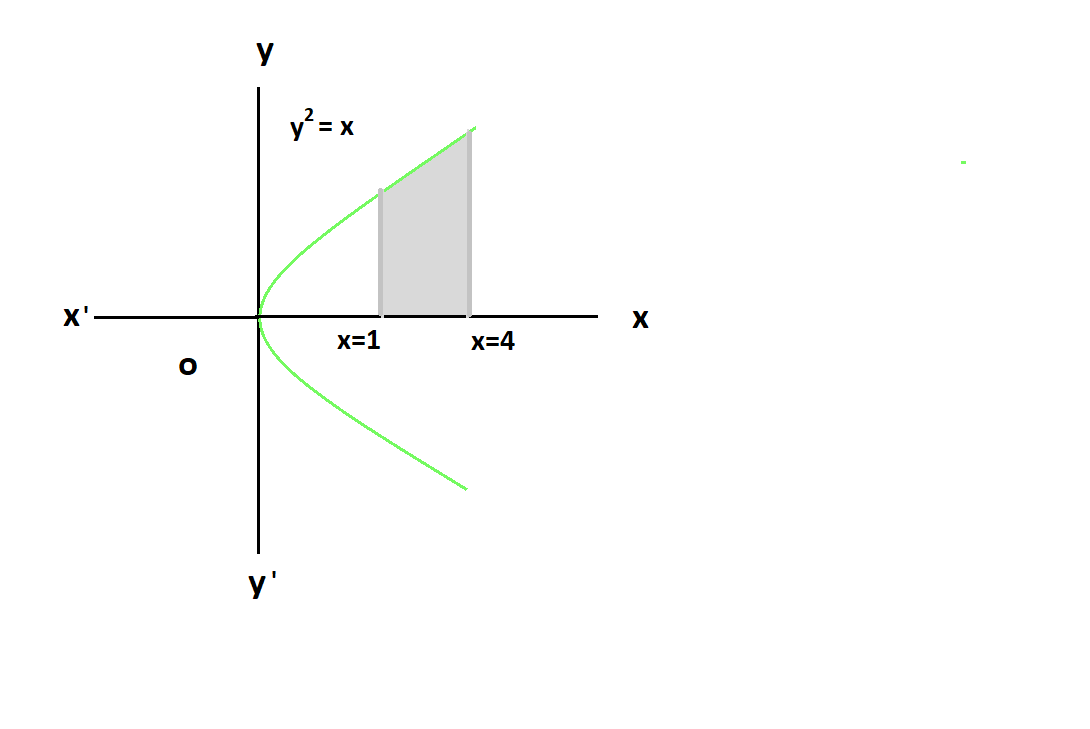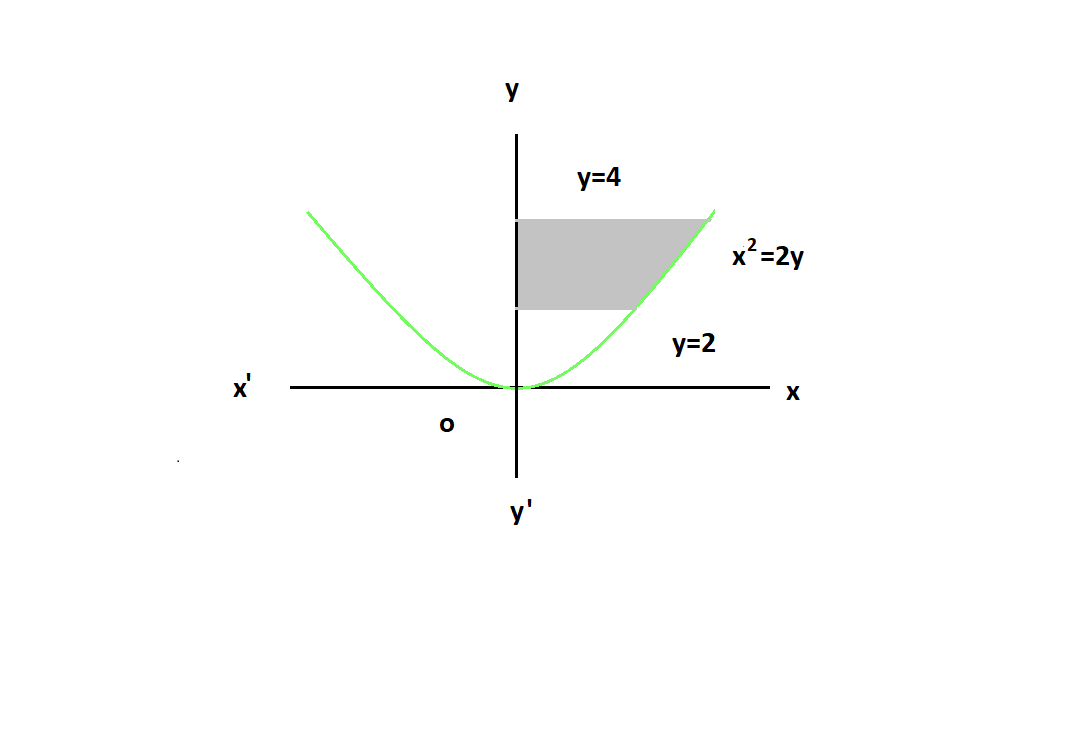Open in App
Not now

# Differentiation and Integration Formula

• Difficulty Level : Basic
• Last Updated : 03 Aug, 2022

Differentiation and Integration both play an important role in mathematics and physics. Without differentiation and integration, we can’t assume mathematics and physics. Both of these are used to find the rate of change in function and things. Differentiation is an instantaneous rate of change and integration is an average rate of change of a function. Both are inverse of each other. Differentiation and Integration are a part of the calculus. There is a fun fact that still, we don’t know how it developed the calculus first by Isaac Newton or Wilhelm Leibniz.

### Differentiation

Differentiation is a method to find the instantaneous rate of change of function or curve. Mathematically, the Slope of the tangent at a point on the curve is called the Derivative of the curve or function. Differentiation is a method to compute the rate at which a dependent variable y changes with respect to the change in the independent variable x. This rate of change is called the derivative of y with respect to x. The concept of Differentiation is also used in physics to derive any expression or to solve a numerical. The opposite of differentiation is called anti- differentiation or Integration.How to Differentiate a function

Procedure

Let’s considered a function f(x),

f(x) = x2

Now differentiating both sides,In differentiation first of all,

We have to put the power of function as the numerator in front of function and reduce the power by 1 of function.

f'(x) = 2x ⇢ (1)

This is usually a step to differentiate the function.

Standard Derivative of a function

• Derivative of Algebraic Function

Quotient Rule: In which situation function or curve in fraction then, we use the Quotient rule to classify.• Derivative of Exponential Function
• Derivative of logarithmic function
• Derivative of Trigonometric Function

### Integration

Integration is a method to find the average rate of change of a function. Integration is a method to find integral. Integral is actually anti-derivative of differentiating function. Differentiation and Integration both are inverse of each other. In simpler words integration is a way to combine all the small components of the system. A system refers to an area that is reserved for any kind of observation or task. We can integrate the function in two ways one is indefinite and the other is definite. In indefinite integration, we get a constant C with our expression but in definite we are able to find the value of that constant C, by restricting its range or limit.

How to Integrate Function

To explain we considered above result i.e. (1) – derivative of function f(x)

f'(x) = 2x

Integrating both sides,

∫f'(x) = ∫2x

Here,

We have to increase the power of derivative by 1 and also divide function with updated power of function,

After that add an integral constant with it.

Integration is called anti-derivativeStandard Integration Function

• Integration  of Algebraic function
• Integration  of Exponential Functions
• Integration of Trigonometric function

### Area under the curve

In our primary classes, we have learned certain formulae of geometry shapes i.e. Square, Triangle, Rectangle, circles, cylinders, and many more and we use those formulae to calculate the area of these various shapes. But there are no such formulae to calculate the area under the curve or enclosed by the curve. but by using the integral calculus concept we can find the area under the curve or area between two lines and also the area of a triangle, square, or other shapes by using definite integrals.

### Sample Problems

Problem 1: Differentiatewith respect to x.

Solution:

LetDifferentiate the y with respect to x.Problem 2: Differentiate the following: i) x3 ii)Solution:

i) Let y = x3ii) LetUsing, Quotient Rule,Problem 3: Find of derivative ofWith respect to x.

Solution:

LetProblem 4: Differentiate with respect to x.

Solution:Problem 5: Differentiate y = Sec2x with respect to x.

Solution:

Let y = sec2x= sec2xtanx

Problem 6: Differentiate sec2x + cos2x.

Solution:

y = sec2x + cos2xProblem 7: Integrate √x with respect to x.

Solution:

y = ∫√x dxdxProblem 8:  Integrate the following : i) e2x ii) eax

Solution:

i) y=∫e2xii) y=∫eaxProblem 9: integrate  Sin2x+ Cos2x.

Solution:

y = ∫sin2x + cos2x

y = ∫dx

y = x + c

Problem 10: integrate sin2x + cos2x.

Solution:

y = ∫sin2x + cos2x

y = ∫sin2x + ∫cos2xProblem 11: Find the area bounded by the curve y = Sinx between x= 0 and x = 2π.

Solution:

Let y = Sinx

The graph of  y = sinx is like,Required area = Area of OABO + Area of BCDB= -cosπ + cos0 + cos2π- cosπ

= 4sq units.

Problem 12: The area bounded by the region of curve y2 = x and the lines x = 1, x= 4, and the x-axis is :

Solution:

Let y2 = x a curve region bounded by the lines x = 1 and x = 4 about x-axis.Required Area (Shaded Area)  =Problem 13: The area of the region area, integrate x w.r.t. y and take y = 2 as the lower limit and y = 4 as the upper limit. The given curve x^2 = 4y is a parabola, which is symmetrical about the y-axis.

Solution:

The given curve is parabola x2 = 4y which is symmetric to the y-axis.The area bounded by the curve is shaded portion of the graph.

Required  Area =My Personal Notes arrow_drop_up
Related Articles